Time: 3 Hours                                                                                                     Max. Marks: 100

NOTE: There are 9 Questions in all.

·      Question 1 is compulsory and carries 20 marks. Answer to Q. 1. A must be written in the space provided for it in the answer book supplied and nowhere else.

·      Out of the remaining EIGHT Questions answer any FIVE Questions. Each question carries 16 marks.

·      Any required data not explicitly given, may be suitably assumed and stated.

Q.1  A   Choose the correct or best alternative in the following:                                         (2x10)

a.       A rectangle has sides 3 cm and 4 cm.  What is the moment of inertia about an axis passing through CG and parallel to the longer side?

(A)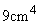(B)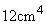(C)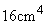(D)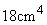b.      Which equation is the correct one, where ‘t’ indicates time, ‘s’ is distance, ‘v’ is velocity and ‘a’ is acceleration

(A)  v.dv = a.dt                                   (B)  v.dv = a.ds

(C)   v.dv = s.da                                  (D)  v.dv = s.dt

c.   A point moves in a curved path starting from rest.  After time ‘t’, it covers a distance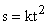and at that instant, the radius of curvature is R, the normal acceleration at that instant is

(A)    2k                                                (B)   2kt

(C)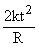(D)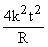d.   A cylinder rolls without slipping on a straight path, the instantaneous centre of rotation will be at

(A)    topmost point of cylinder.            (B) bottommost point of the cylinder.

(C)  centre of cylinder.                        (D)  at a height of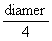from bottom.

e.   The property of the bodies by virtue of which they rebound after impact is called.

(A)     plasticity.                                      (B)  elasticity

(C)  ductility.                                       (D)  hardness.

f.    Which cross section of a steel beam of minimum weight is capable of with- standing a concentrated load at the centre?

(A)     circular                                         (B)  hollow circular

(C)  rectangular                                   (D)  I-section

g.   All cross sections of a wire of close coiled helical spring which is axially loaded are subjected to

(A)    tensile stress                                 (B) torsional shear stress

(C) transverse shear stress                   (D) both torsional shear and transverse

shear stresses.

h.   When a floating body is given a small angular displacement, it starts oscillating about a point which is known as:

(A)  centre of pressure                         (B)  meta-centre

(C)  centre of buoyancy                       (D)  centre of gravity

i.    The frictional factor for a turbulent flow in a smooth pipe varies as

(A)  Re                                                (B)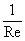(C)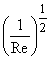(D)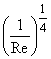[where Re is Reynolds number]

j.    Which of the following functions represent the velocity potential of fluid flow:

(A)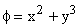(B)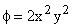(C)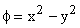(D)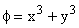Answer any FIVE Questions out of EIGHT Questions.

Each question carries 16 marks.

Q.2     a.   Define moment of inertia.                                                                                    (2)

b.   Find the moment of inertia of the figure shown in Fig.1 about

(i)   an axis passing through centroid and parallel to AB.

(ii)  and axis passing though centroid and parallel to AC.                                   (14)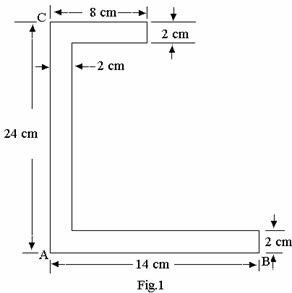Q.3      a.   State Newton’s second law.                                                                               (2)

b.   An aeroplane is flying horizontally at a height of 800 m with a speed of 1000 Km/hr sees a target at a distance of 2500 m on the ground and drops a bomb.  Does the bomb hit the target or misses it?  What is the time taken by the bomb to reach the ground?                                                                (14)

Q.4       a.   A body of mass ‘m’ is rotating about an axis.  State the Newton’s laws of rotation.            (3)

b.   A train weighing 20,000 kN moves down a slope of 1 in 150 at 18 km/hr and engine develops 350 kW.  What power will be required to pull the train up the slope at the same speed?                     (13)

Q.5       a.   Define amplitude and frequency.                                                                         (4)

b.   A particle is moving with simple harmonic motion and performs 8 complete oscillations per minute.  If the particle is 5 cm from the centre of oscillation, find the amplitude, the velocity of the particle and the maximum acceleration.  Given that the velocity of particle at a distance of 7 cm from the centre of oscillation is 0.6 times the maximum velocity.                 (12)

Q.6     a.   What is a point of contra flexure?                                                                       (2)

b.   A beam AB, one metre long carries a uniformly distributed load of 2000 N/m.  The beam is supported at the left end A and at a point C which is at 0.3 m from the end B.  Find the value of distance ‘x’ from the end B, where bending moment is zero. Draw the bending moment and shear force diagrams for this arrangement.                                                              (14)

Q.7     a.   Define longituduial stress and hoop stress in a cylinder.                                        (4)

b.   Calculate the increase in volume of a boiler shell 2.5 m long, 1m diameter, when it is subjected to an internal pressure of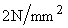.  The maximum tensile stress is limited to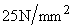under the above pressure.  Find the thickness of the shell.  Take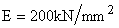and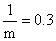.           (12)

Q.8     a.   Write the components of acceleration in x, y and z directions at any point in a fluid flow.                  (3)

b.      The inlet and throat diameters of a horizontal venturimeter are 30cm and 10 cm respectively.  The liquid flowing is water.  The pressure intensity at inlet is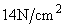while the vacuum pressure head at the throat is 37 cm of mercury.  Assume that 4% of the differential head is lost between the inlet and throat.  Find the coefficent of venturimeter and rate of flow.                                                        (13)

Q.9     a.   Define mechanical efficiency of a turbine.                                                            (2)

b.   A Pelton wheel is required to develop 6000 kW when working under a head of 300 m.  It rotates with a speed of 550 rpm.  Assuming jet ratio as 10 and overall efficiency of 86%.  Calculate

(i)                  diameter of wheel

(ii)                quantity of water required

(iii)               no. of jets

Assume velocity coefficient = 0.98 and speed ratio = 0.46.                              (14)

OR

Writes short note on:

(i)                  Free body diagram with examples.

(ii)                   Streamlines, rotational flow & turbulence.

(iii)                 Continuity Equation.

(iv)                 Flow around cylindrical body.                                        (4x4=16)